GeeksforGeeks App
Open AppBrowser
Continue

# Solving Absolute Value Equations

The concept of absolute values comes under the parent topic- Algebra. It is one of the broad areas of mathematics. It deals with the study of mathematical symbols and rules for manipulating the symbols. In general, the concept of absolute values is used at distances to represent the distance between any two points.

Example: |-7| = 7, |7| = 7, |0| = 0

The result of absolute value is always positive. It won’t be negative.

### Solving Absolute Value Equations

Absolute Value Equations are equations where the variable is between absolute value operators. Example: |x-2| = 0. Solving absolute value equations can be explained better by taking an example and solving those to get a better understanding.

Question 1: Solve the equation |x + 4| = 1 such that what are the possible values of x.

Solution:

Step: 1 First we need to write two equations from the given equation such a way that

x + 4 = 1

x + 4 = -1

Step: 2 Solve the above formed equations to get possible values of x

x + 4 = 1

x = 1 – 4

x = -3

x + 4 = -1

x = -1 – 4

x = -5

Step: 3 Verify the above results by substituting the x values in the given equation

|x + 4| = 1

Substitute x = -3 in the above equation

|-3 + 4| = 1

|4 – 3| = 1

1 = 1

Hence x = -3 satisfies the given equation.

|x + 4| = 1

Substitute x = -5 in the above equation

|-5 + 4| = 1

|4 – 5| = 1

|-1| = 1

1 = 1

Hence x = -5 satisfies the given equation.

So, the possible values of x are -3, -5.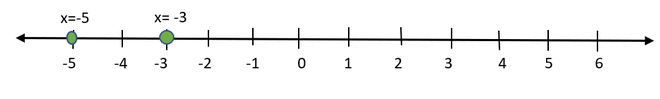### Steps to solve absolute value equations

From the above example, we can derive steps to solve the absolute value equations,

1. Write two equations from the given equation as per the above example.
2. Solve the equations formed from step-1 to get possible values of the variable.
3. Verify the results we got from step-2 in the given equation.

Let’s look at different types of scenarios/equations/problems to solve.

### Sample Problems

Question 1: Solve |2x – 5| = 9

Solution:

Step-1 Make 2 equations from given equation

2x – 5 = 9

2x – 5 = -9

Step-2 Solve the above equations

2x = 9 + 5

2x = 14

x = 14/2

x = 7

2x = -9 + 5

2x = -4

x = -2

Step-3 Verify the above results by substituting in the given equation

Given equation |2x – 5| = 9

Substitute x = 7 in given equation

|2(7) – 5| = 9

|14 – 5| = 9

9 = 9

Substitute x = -2 in given equation

|2(-2) – 5| = 9

|-4 – 5| = 9

9 = 9

Hence x = 7, x = -2 are the possible values.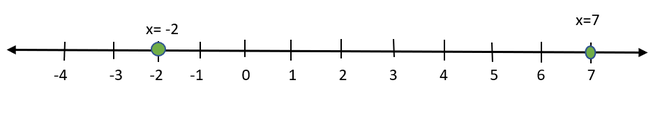Question 2: Solve the expression 2 |x + 1| – 2 = 4

Solution:

In order to proceed to step-1 we can simplify the equation such that left side only has absolute value function and right side of equal to is a value.

2 |x + 1| -2 = 4

2 |x + 1| = 4 + 2

2 |x + 1| = 6

|x + 1| = 6/2

|x + 1| = 3

This equation is used to write two equations,

Step-1:

x + 1 = 3

x + 1 = -3

Step-2: Solve the above equations

x = 3 – 1

x = 2

x = -3 – 1

x = -4

Step-3: Verification

2 |x + 1| – 2 = 4

Substitute x = 2 in the given equation

2 |2 + 1| – 2 = 4

2 |3| – 2 = 4

6 – 2 = 4

4 = 4

Substitute x = -4 in the given equation

2 |-4 + 1| – 2 = 4

2 |-3| – 2 = 4

2 (3) -2 = 4

6 – 2 = 4  => 4 = 4

So the possible values of x after solving the equation 2 |x + 1| – 2 = 4 are 2, -4.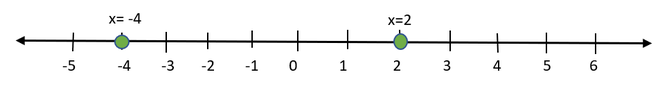Question 3: Solve |2x – 5| = -1.

Solution:

Note: The absolute value function can never be equal to negative value.

For the given equation where the result is negative which indicates that there is no solution for this equation.

Question 4: Solve |2x – 5| = x + 4

Solution:

Step-1 Write 2 equations from the above equation

2x – 5 = x + 4

2x – 5 = -(x + 4)

Step-2 Solve these equations

2x – x = 5 + 4

x = 9

2x – 5 = -x – 4

2x + x = 5 – 4

3x = 1

x = 1/3

Step-3 Verification

Given equation, |2x – 5| = x + 4

Substitute x = 9 in the given equation

|2(9) – 5| = 9 + 4

|18 – 5| = 13

|13| =13

13 = 13

Substitute x=(1/3) in the given equation

|2(1/3) – 5| = (1/3) + 4

|(2/3) – 5| = (1/3) + 4

|(2 – 15)/3| = (1 + 12)/3

|-13/3| = 13/3 =>13/3 =13/3

So the possible values of x after solving the equation |2x – 5| = x + 4 are 9, 1/3.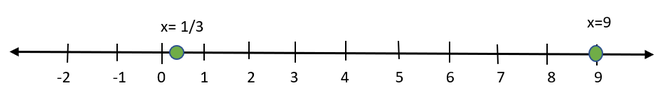Question 5: Solve |7 + 7x| = -8

Solution:

There will be no way to solve the above equation because the result of absolute value equation will never be negative. In this equation the resultant value of absolute value equation is negative. So no solution for this equation.

Question 6: Solve |4t – 2| = |8t + 18|

Solution:

Step-1 Write 2 equations from the above equation

4t – 2 = -(8t + 18)

4t – 2 = 8t + 18

Step-2 Solve these equations

4t – 2 = -8t – 18

4t + 8t = -18 + 2

12t = -16

t = -16/12

t = -4/3

4t – 8t = 18 + 2

-4t = 20

t = -20/4

t = -5

Step-3 Verification

Substitute t = -4/3 in the given equation

|4(-4/3) – 2| = |8(-4/3) + 18|

|(-16/3) – 2| = |(-32/3) +18|

|(-16 – 6)/3| = |(-32 + 54)/3|

|-22/3| = |22/3|

22/3 = 22/3

Substitute t = -5 in the given equation

|4(-5) – 2| = |8(-5) + 18|

|-20 – 2| = |-40 + 18|

|-22| = |-22|

22=22

So the possible values of t for the given absolute value equation are -4/3 & -5.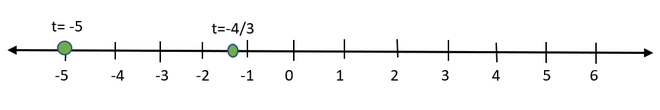My Personal Notes arrow_drop_up
Related Tutorials